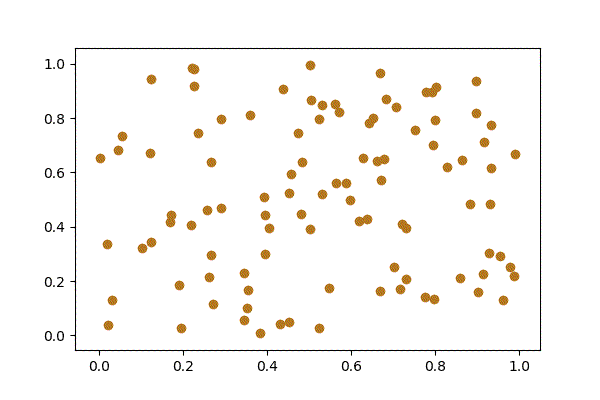# pyrecorder¶# Installation¶

The framework is available at the PyPi Repository:

pip install -U pyrecorder


# Output¶

## GIF¶

:

import numpy as np
import matplotlib.pyplot as plt

from pyrecorder.video import Video
from pyrecorder.recorders.gif import GIF

vid = Video(GIF("example.gif"))

for k in range(10):
X = np.random.random((100, 2))
plt.scatter(X[:, 0], X[:, 1])
vid.record()

vid.close()


Now you can check out the GIF file or also render it in Jupyter with

from pyrecorder.video import load
load("example.gif")


The resulting animation looks as follows:

:## Video¶

:

import numpy as np
import matplotlib.pyplot as plt

from pyrecorder.video import Video
from pyrecorder.recorders.file import File

vid = Video(File("example.mp4"))

for k in range(10):
X = np.random.random((100, 2))
plt.scatter(X[:, 0], X[:, 1])
vid.record()

vid.close()


The file has been stored to the provided path. You can also render the video in Jupyter with

from pyrecorder.video import load
load("example.mp4")


The resulting animation looks as follows:

:


## Converter¶

### Matplotlib¶

Examples are shown above.

:

import numpy as np
import matplotlib.pyplot as plt

from pyrecorder.converters.matplotlib import Matplotlib
from pyrecorder.recorders.file import File
from pyrecorder.video import Video

fname = "example_matplotlib.mp4"

with Video(File(fname), converter=Matplotlib()) as vid:

for k in range(10):
fig, (ax1, ax2) = plt.subplots(2)

X = np.random.random((100, 2))
ax1.scatter(X[:, 0], X[:, 1], color="green")

X = np.random.random((100, 2))
ax2.scatter(X[:, 0], X[:, 1], color="red")

vid.record(fig=fig)

: